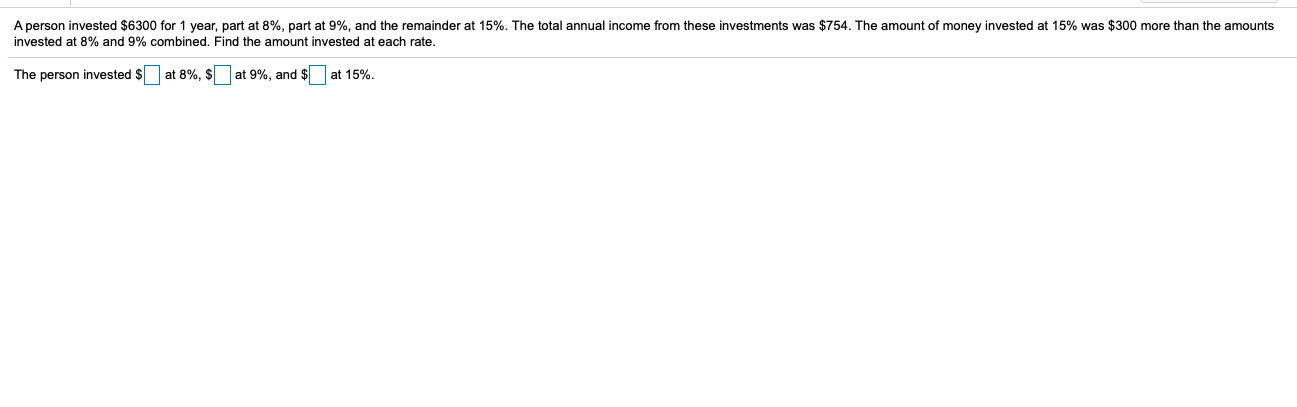# A person invested $6300 for 1 year, part at 8%, part at 9%, and the remainder at 15%. The total annual income from these investments was$754. The amount of money invested at 15% was $300 more than the amounts invested at 8% and 9% combined, Find the amount invested at each rate. The person invested$ at 8%, $at 9%, and$ at 15%.

Questionhelp_outlineImage TranscriptioncloseA person invested $6300 for 1 year, part at 8%, part at 9%, and the remainder at 15%. The total annual income from these investments was$754. The amount of money invested at 15% was $300 more than the amounts invested at 8% and 9% combined, Find the amount invested at each rate. The person invested$ at 8%, $at 9%, and$ at 15%. fullscreen

### Want to see this answer and more?

Experts are waiting 24/7 to provide step-by-step solutions in as fast as 30 minutes!*

*Response times vary by subject and question complexity. Median response time is 34 minutes and may be longer for new subjects.
Tagged in
MathAlgebra

### Applications of Mathematics